# 线性回归中的方差齐性探察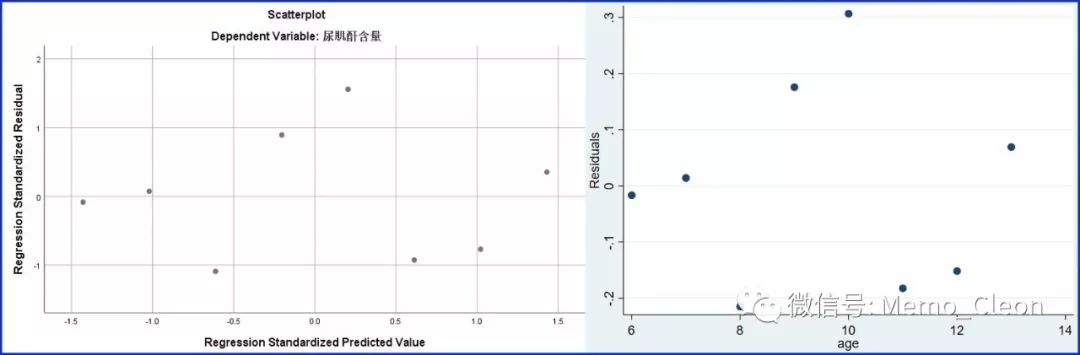use "D:TempsLR.dta"

regress Uc age

rvpplot age

SPSS中仅提供了线性回归等方差判断的图示法，统计学检验方法可在STATA、R等软件中实现。

STATA检验步骤：统计>>线性模型及相关>>回归诊断>>模型检定等。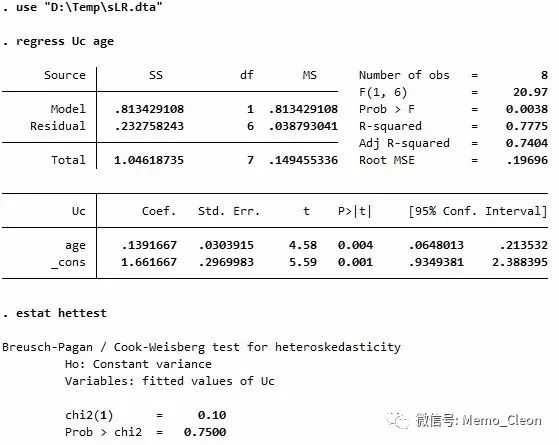estat hettest performs three versions of the Breusch-Pagan (1979) and Cook-Weisberg (1983) test for heteroskedasticity. All three versions of this test present evidence against the null hypothesis that t=0 in Var(e)=sigma^2 exp(zt). In the normal version, performed by default, the null hypothesis also includes the assumption that the regression disturbances are independent-normal draws with variance sigma^2.  The normality assumption is dropped from the null hypothesis in the iid and fstat versions, which respectively produce the score and F tests discussed in Methods and formulas in [R] regress postestimation.  If varlist is not specified, the fitted values are used for z.  If varlist or the rhs option is specified, the variables specified are used for z.

setwd("D:/Temp")  #设置工作目录#

library(foreign)  #加载foreign程序包#

var<-lm(Uc~age,data=normvar)  #以age拟合Uc的直线回归#

summary(var)  #显示拟合模型的详细结果#

library(lmtest)  #加载lmtest程序包#

library(zoo)  #加载zoo程序包#

bptest(var)  #Breusch-Pagan检验#

library(car)  #加载car程序包#

library(carData)  #加载carData程序包#

ncvTest(var)  #得分检验残差是否恒定#

library(gvlma)  #加载gvlma程序包#

gvlma(var)  #线性模型假设的综合检验#

①模型拟合与SPSS结果完全一致：截距为1.66，age系数为0.14，均有统计学意义；决定系数0.78，校正决定系数0.74，加入变量age后，模型的改变有统计学意义（P=0.004）。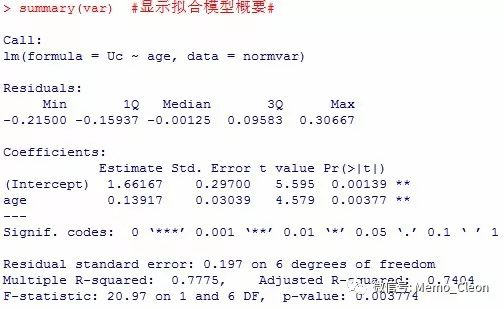Breusch-Pagan检验结果：P=0.6536>0.05，方差是不变的（相等的），满足方差齐性。

studentized Breusch-Pagan test

data:  var

BP = 0.20142, df = 1, p-value = 0.6536

Non-constant Variance Score Test

Variance formula: ~ fitted.values

Chisquare = 0.1015182, Df = 1, p = 0.75002

Suggested power transformation:  -5.02297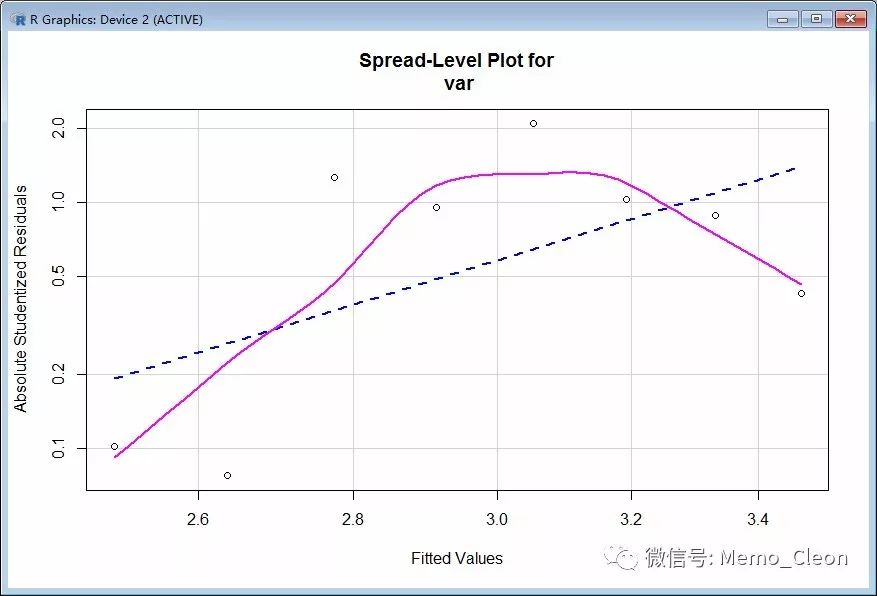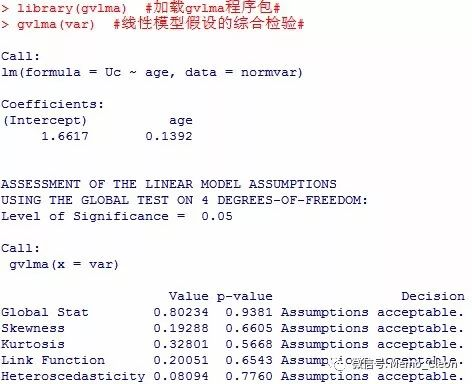• 版权声明 本文源自 一统浆糊 整理 发表
• 转载请务必保留本文链接：https://www.plob.org/article/23842.html